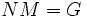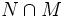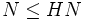# Normal Hall implies permutably complemented

This article gives the statement and possibly, proof, of an implication relation between two subgroup properties. That is, it states that every subgroup satisfying the first subgroup property (i.e., normal Hall subgroup) must also satisfy the second subgroup property (i.e., permutably complemented subgroup)
View all subgroup property implications | View all subgroup property non-implications
Get more facts about normal Hall subgroup|Get more facts about permutably complemented subgroup

## Name

This result is sometimes called Schur's theorem, and is considered a part of the Schur-Zassenhaus theorem, which also asserts that any two permutable complements to a normal Hall subgroup are conjugate. The second half is available at Hall retract implies order-conjugate.

## Statement

### Statement with symbols

Suppose$N$ is a normal Hall subgroup of a group$G$. Then there exists a subgroup$H \le G$ such that$N \cap H$ is trivial and$NH = G$. Note that this makes$H$ a Hall subgroup as well.

Equivalently, if$N$ is a normal$\pi$-Hall subgroup of$G$, then$G$ possesses a$\pi'$-Hall subgroup: a Hall subgroup corresponding to the primes not in$\pi$.

## Proof

We prove this claim by induction on the order of$G$. Rather, we reduce the claim to (1) by induction on the order of$G$.

Given: A group$G$, a normal Hall subgroup$N$ of$G$.

To prove:$N$ has a permutable complement in$G$.

Proof: Note that for any prime$p$ dividing the order of$N$, a$p$-Sylow subgroup of$N$ is also$p$-Sylow in$G$. Note also that by fact (2),$p$-Sylow subgroups exist for all primes$p$.

1. Case where there is a prime$p$ dividing the order of$N$, such that a$p$-Sylow subgroup$P$ of$N$ is not normal in$G$: Let$M = N_G(P)$.$NM = G$ by fact (3) (Frattini's argument). Further, by fact (4),$N \cap M$ is a normal Hall subgroup of$M$, so by induction, there exists a subgroup$H$ of$M$ that is a permutable complement in$M$ to$N \cap M$. We claim that$H$ is a permutable complement to$N$ in$G$:
•$H \cap N$ is trivial: Since$H \le M$ and$H \cap (N \cap M)$ is trivial, we obtain that$H \cap N$ is trivial.
•$HN = G$: We have$M = H(N \cap M) \le HN$. Also,$N \le HN$. Thus,$NM \le HN$. But as observed earlier, by fact (3),$NM = G$. this forces$HN = G$.
2. Case where$N$ is non-abelian and, for every prime$p$ dividing the order of$N$, a$p$-Sylow subgroup of$N$ is normal in$G$: In this case, each of the$p$-Sylow subgroups of$N$ is normal in$N$, hence$N$ is a direct product of its Sylow subgroups. Thus,$N$ is nilpotent. In particular, the center$Z(N)$ is nontrivial. By fact (6),$Z(N)$ is normal in$G$. Consider the group$G_1 = G/Z(N)$.$N_1 = N/Z(N)$ is a normal Hall subgroup of$G_1$, so by induction, there exists a permutable complement$H_1$ to$N_1$ in$G_1$. Suppose$K$ is the inverse image of$H_1$ under the quotient map$G \to G/Z(N)$. Note that$K$ is a proper subgroup of$G$, since$Z(N)$ is a proper subgroup of$N$ by the assumption that$N$ is not abelian.$K/Z(N)$ and$Z(N)$ have relatively prime orders, so$Z(N)$ is a normal Hall subgroup of$K$. Thus, there exists a permutable complement$H$ to$Z(N)$ in$K$.
3. Case that$N$ is abelian: This is covered by fact (1).